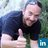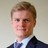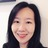Successfully reported this slideshow.Upcoming SlideShare
×

ofUpcoming SlideShare
Next

4 Likes

Share

# Cardinality Estimation through Histogram in Apache Spark 2.3 with Ron Hu and Zhenhua Wang

Apache Spark 2.2 shipped with a state-of-art cost-based optimization framework that collects and leverages a variety of per-column data statistics (e.g., cardinality, number of distinct values, NULL values, max/min, avg/max length, etc.) to improve the quality of query execution plans. Skewed data distributions are often inherent in many real world applications. In order to deal with skewed distributions effectively, we added equal-height histograms to Apache Spark 2.3. Leveraging reliable statistics and histogram helps Spark make better decisions in picking the most optimal query plan for real world scenarios.

In this talk, we’ll take a deep dive into how Spark’s Cost-Based Optimizer estimates the cardinality and size of each database operator. Specifically, for skewed distribution workload such as TPC-DS, we will show histogram’s impact on query plan change, hence leading to performance gain.

See all

### Cardinality Estimation through Histogram in Apache Spark 2.3 with Ron Hu and Zhenhua Wang

1. 1. Ron Hu, Zhenhua Wang Huawei Technologies, Inc. Cardinality Estimation through Histogram in Apache Spark 2.3 #DevSAIS13
2. 2. Agenda • Catalyst Architecture • Cost Based Optimizer in Spark 2.2 • Statistics Collected • Histogram Support in Spark 2.3 • Configuration Parameters • Q & A 2
3. 3. Catalyst Architecture 3 Spark optimizes query plan here Reference:Deep Dive into Spark SQL’s Catalyst Optimizer, a databricks engineering blog
4. 4. Query Optimizer in Spark SQL • Spark SQL’s query optimizer is based on both rules and cost. • Most of Spark SQL optimizer’s rules are heuristics rules. – PushDownPredicate, ColumnPruning, ConstantFolding,…. • Cost based optimization (CBO) was added in Spark 2.2. 4
5. 5. Cost Based Optimizer in Spark 2.2 • It was a good and working CBO framework to start with. • Focused on – Statistics collection, – Cardinality estimation, – Build side selection, broadcast vs. shuffled join, join reordering, etc. • Used heuristics formula for cost function in terms of cardinality and data size of each operator. 5
6. 6. Statistics Collected • Collect Table Statistics information • Collect Column Statistics information • Goal: – Calculate the cost for each operator in terms of number of output rows, size of output, etc. – Based on the cost calculation, adjust the query execution plan 6
7. 7. Table Statistics Collected • Command to collect statistics of a table. – Ex: ANALYZE TABLE table-name COMPUTE STATISTICS • It collects table level statistics and saves into metastore. – Number of rows – Table size in bytes 7
8. 8. Column Statistics Collected • Command to collect column level statistics of individual columns. – Ex: ANALYZE TABLE table-name COMPUTE STATISTICS FOR COLUMNS column-name1, column-name2, …. • It collects column level statistics and saves into meta-store. String/Binary type ✓ Distinct count ✓ Null count ✓ Average length ✓ Max length Numeric/Date/Timestamp type ✓ Distinct count ✓ Max ✓ Min ✓ Null count ✓ Average length (fixed length) ✓ Max length (fixed length) 8
9. 9. Real World Data Are Often Skewed 9#DevSAIS13 – Cardinality Estimation by Hu and Wang
10. 10. Histogram Support in Spark 2.3 • Histogram is effective in handling skewed distribution. • We developed equi-height histogram in Spark 2.3. • Equi-Height histogram is better than equi-width histogram • Equi-height histogram can use multiple buckets to show a very skewed value. • Equi-width histogram cannot give right frequency when a skewed value falls in same bucket with other values. Column interval Frequency Equi-Width Equi-Height Column interval Frequency Density 10
11. 11. Histogram Algorithm – Each histogram has a default of 254 buckets. • The height of a histogram is number of non-null values divided by number of buckets. – Each histogram bucket contains • Range values of a bucket • Number of distinct values in a bucket – We use two table scans to generate the equi-height histograms for all columns specified in analyze command. • Use ApproximatePercentile class to get end points of all histogram buckets • Use HyperLogLog++ algorithm to compute the number of distinct values in each bucket. 11
12. 12. Filter Cardinality Estimation • Between Logical expressions: AND, OR, NOT • In each logical expression: =, <, <=, >, >=, in, etc • Current support type in Expression – For <, <=, >, >=, <=>: Integer, Double, Date, Timestamp, etc – For = , <=>: String, Integer, Double, Date, Timestamp, etc. • Example: A <= B – Based on A, B’s min/max/distinct count/null count values, decide the relationships between A and B. After completing this expression, we set the new min/max/distinct count/null count – Assume all the data is evenly distributed if no histogram information. 12
13. 13. Filter Operator without Histogram • Column A (op) literal B – (op) can be “=“, “<”, “<=”, “>”, “>=”, “like” – Column’s max/min/distinct count/null count should be updated – Example: Column A < value B Column AB B A.min A.max Filtering Factor = 0% need to change A’s statistics Filtering Factor = 100% no need to change A’s statistics Filtering Factor = (B.value – A.min) / (A.max – A.min) A.min = no change A.max = B.value A.ndv = A.ndv * Filtering Factor 13 • Without histogram, we prorate over the entire column range. • It works only if it is evenly distributed.
14. 14. Filter Operator with Histogram • With histogram, we check the range values of a bucket to see if it should be included in estimation. • We prorate only the boundary bucket. • This way can enhance the accuracy of estimation since we prorate (or guess) only a much smaller set of records in a bucket only. 14
15. 15. Histogram for Filter Example 1 Age distribution of a restaurant: • Estimate row count for predicate “age > 40”. Correct answer is 5. • Without histogram, estimate: 25 * (80 – 40)/(80 – 20) = 16.7 • With histogram, estimate: 1.0 * // only 5th bucket 5 // 5 records per bucket = 5 15#DevSAIS13 – Cardinality Estimation by Hu and Wang 20 21 23 24 25 ndv=5 25 27 27 27 28 ndv=3 28 28 28 28 28 ndv=1 29 36 36 39 40 ndv=4 45 47 55 63 80 ndv=5 20 25 28 40 8028 Total row count: 25 age min = 20 age max = 80 age ndv = 17
16. 16. Histogram for Filter Example 2 Age distribution of a restaurant: • Estimate row count for predicate “age = 28”. Correct answer is 6. • Without histogram, estimate: 25 * 1 / 17 = 1.47 • With histogram, estimate: ( 1/3 // prorate the 2nd bucket + 1.0 // for 3rd bucket ) * 5 // 5 records per bucket = 6.67 16#DevSAIS13 – Cardinality Estimation by Hu and Wang 20 21 23 24 25 ndv=5 25 27 27 27 28 ndv=3 28 28 28 28 28 ndv=1 29 36 36 39 40 ndv=4 45 47 55 63 80 ndv=5 20 25 28 40 8028 Total row count: 25 age min = 20 age max = 80 age ndv = 17
17. 17. Join Cardinality without Histogram • Inner-Join: The number of rows of “A join B on A.k1 = B.k1” is estimated as: num(A ⟗ B) = num(A) * num(B) / max(distinct(A.k1), distinct(B.k1)), – where num(A) is the number of records in table A, distinct is the number of distinct values of that column. – The underlying assumption for this formula is that each value of the smaller domain is included in the larger domain. – Assuming uniform distribution for entire range of both join columns. • We similarly estimate cardinalities for Left-Outer Join, Right-Outer Join and Full-Outer Join 17
18. 18. Join Cardinality without Histogram 18 Total row count: 25 k1 min = 20 k1 max = 80 k1 ndv = 17 Table A, join column k1 Table B, join column k1 Total row count: 20 k1 min = 20 k1 max = 90 k1 ndv = 17 Without histogram, join cardinality estimate is 25 * 20 / 17 = 29.4 The correct answer is 20. 20 21 23 24 25 25 27 27 27 28 28 28 28 28 28 29 36 36 39 40 45 47 55 63 80 20 80 20 21 21 25 26 28 28 30 36 39 45 50 55 60 65 70 75 80 90 90 20 90
19. 19. Join Cardinality with Histogram • The number of rows of “A join B on A.k1 = B.k1” is estimated as: num(𝐴⟗𝐵) = 𝑖,𝑗 num(𝐴𝑖) * num(𝐵𝑗) / max (ndv(Ai.k1), ndv(Bj.k1)) – where num(Ai) is the number of records in bucket i of table A, ndv is the number of distinct values of that column in the corresponding bucket. – We compute the join cardinality bucket by bucket, and then add up the total count. • If the buckets of two join tables do not align, – We split the bucket on the boundary values into more than 1 bucket. – In the split buckets, we prorate ndv and bucket height based on the boundary values of the newly split buckets by assuming uniform distribution within a given bucket. 19
20. 20. Aligning Histogram Buckets for Join • Form new buckets to align buckets properly 20#DevSAIS13 – Cardinality Estimation by Hu and Wang Table A, join column k1, Histogram buckets Table B, join column k1, Histogram buckets 20 25 30 50 70 9080 28 28 40 Original bucket boundary Extra new bucket boundary To form additional buckets This bucket is excluded In computation 20 25 28 28 40 80705030
21. 21. 21#DevSAIS13 – Cardinality Estimation by Hu and Wang Table A, join column k1, Histogram buckets: Total row count: 25 min = 20, max = 80 ndv = 17 20 21 23 24 25 ndv=5 25 27 27 27 28 ndv=3 28 28 28 28 28 ndv=1 29 ndv=1 36 36 39 40 ndv=3 45 47 ndv=2 55 63 ndv=2 80 ndv=1 2520 28 3028 5040 70 80 90 90 ndv=1 20 21 21 25 ndv=3 26 ndv=1 28 28 ndv=1 30 ndv=1 36 39 ndv=2 45 50 ndv=2 55 60 65 70 ndv=4 75 80 ndv=2 7030282520 28 5040 80 90 Table B, join column k1, Histogram buckets: Total row count: 20 min = 20, max = 90 ndv = 17 - With histogram, join cardinality estimate is 21.8 by computing the aligned bucket’s cardinality one-by-one. - Without histogram, join cardinality estimate is 29.4 - The correct answer is 20.
22. 22. Other Operator Estimation • Project: does not change row count • Aggregate: consider uniqueness of group-by columns • Limit, Sample, etc. 22
23. 23. Statistics Propagation Join (t1.a = t2.b) Scan t2Scan t1a: min, max, ndv … … b: min, max, ndv … … a: newMin, newMax, newNdv … b: newMin, newMax, newNdv … … Top-down statistics requests Bottom-up statistics propagation 23
24. 24. Statistics inference • Statistics collected: – Number of records for a table – Number of distinct values for a column • Can make these inferences: – If the above two numbers are close, we can determine if a column is a unique key. – Can infer if it is a primary-key to foreign-key join. – Can detect if a star schema exists. – Can help determine the output size of group-by operator if multiple columns of same tables appear in group-by expression. 24
25. 25. Configuration Parameters Configuration Parameters Default Value Suggested Value spark.sql.cbo.enabled False True spark.sql.cbo.joinReorder.enabled False True spark.sql.cbo.joinReorder.dp.threshold 12 12 spark.sql.cbo.joinReorder.card.weight 0.7 0.7 spark.sql.statistics.size.autoUpdate.enabled False True spark.sql.statistics.histogram.enabled False True spark.sql.statistics.histogram.numBins 254 254 spark.sql.statistics.ndv.maxError 0.05 0.05 spark.sql.statistics.percentile.accuracy 10000 10000 25#DevSAIS13
26. 26. Reference • SPARK-16026: Cost-Based Optimizer Framework – https://issues.apache.org/jira/browse/SPARK-16026 – It has 45 sub-tasks. • SPARK-21975: Histogram support in cost-based optimizer – https://issues.apache.org/jira/browse/SPARK-21975 – It has 10 sub-tasks. 26#DevSAIS13 – Cardinality Estimation by Hu and Wang
27. 27. Summary • Cost Based Optimizer in Spark 2.2 • Statistics Collected • Histogram Support in Spark 2.3 – Skewed data distributions are intrinsic in real world data. – Turn on histogram configuration parameter “spark.sql.statistics.histogram.enabled” to deal with skew. 27
28. 28. Q & A ron.hu@huawei.com wangzhenhua@huawei.com
•#### meraboxer

Mar. 16, 2021
•#### AsmaZgolli

Sep. 12, 2019
•#### nickchervov

Sep. 2, 2018
•#### soyoungyu

Jul. 21, 2018

Total views

2,211

On Slideshare

0

From embeds

0

Number of embeds

10

81

Shares

0